Concepts

Class 10
Chapter 4 Class 10 - Carbon and its Compounds

## What are Isomers?

When two or more compounds having the same formula but different structures are called isomers.

### Example 1

n-butane and Isobutane both have the same chemical  formula C 4 H 10 .But they have different structure . .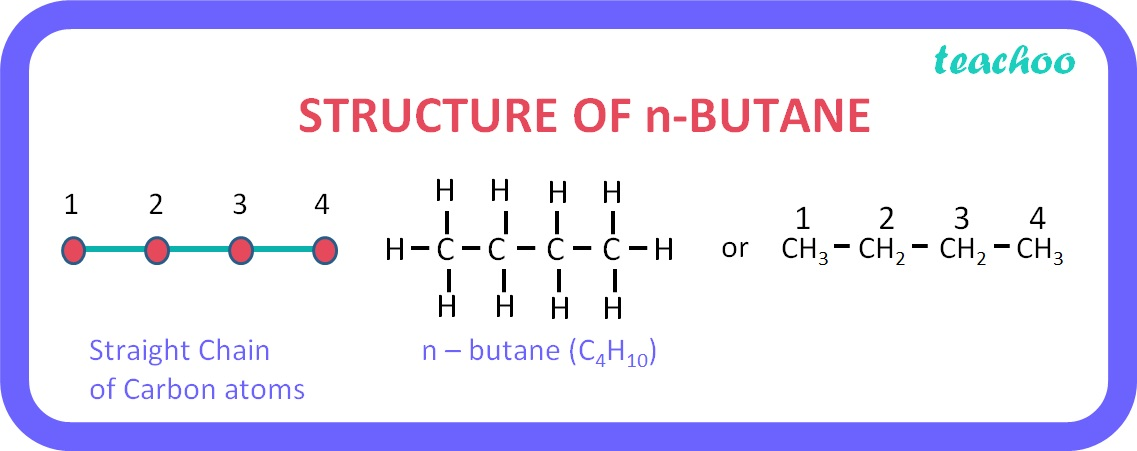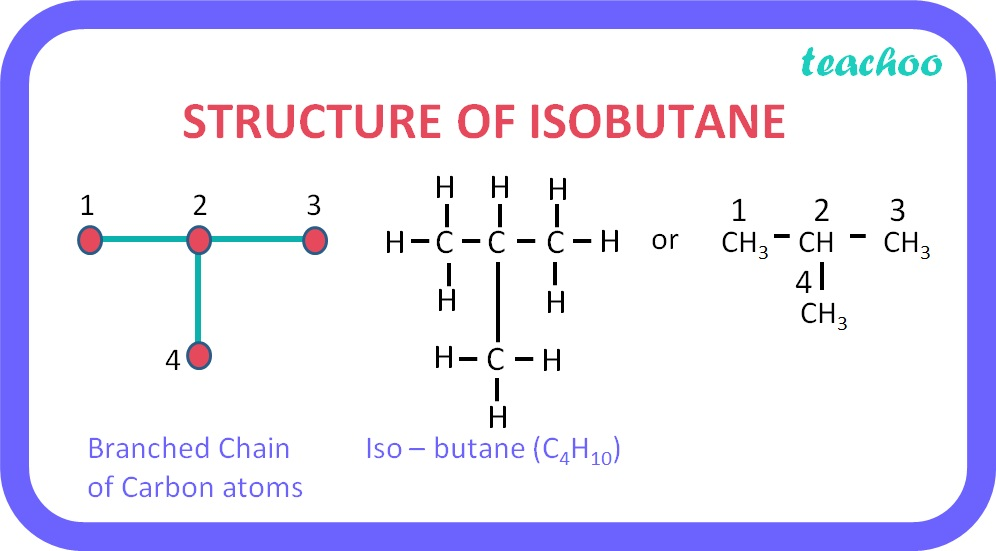### Explanation for n-butane

• n-butane means Normal Butane
• Formula for normal butane is C 4 H 10
• ‘but’ stands for 4, hence it has 4 carbon atoms
• Number of hydrogen atoms = 2 × Carbon Atoms + 2 = 2 × 4 + 2 = 8 + 2 = 10

Hence we get formula C 4 H 10

In n butane, we show all 4 carbon atoms together as shown below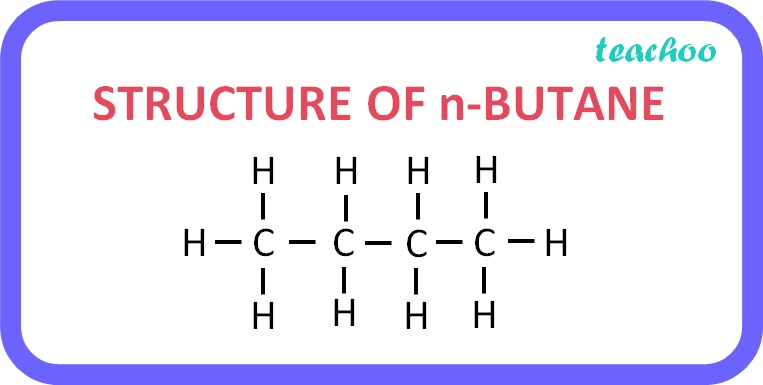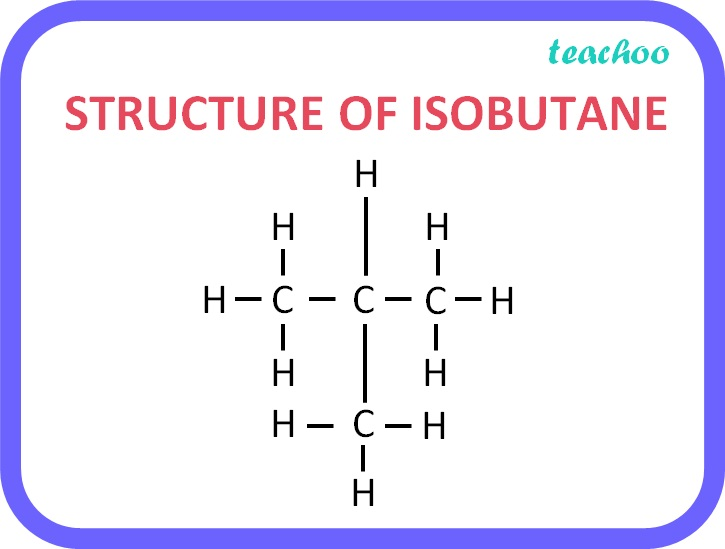### Example 2

n-pentane, Isopentane and neopentane all have formula C5H12, but different structures.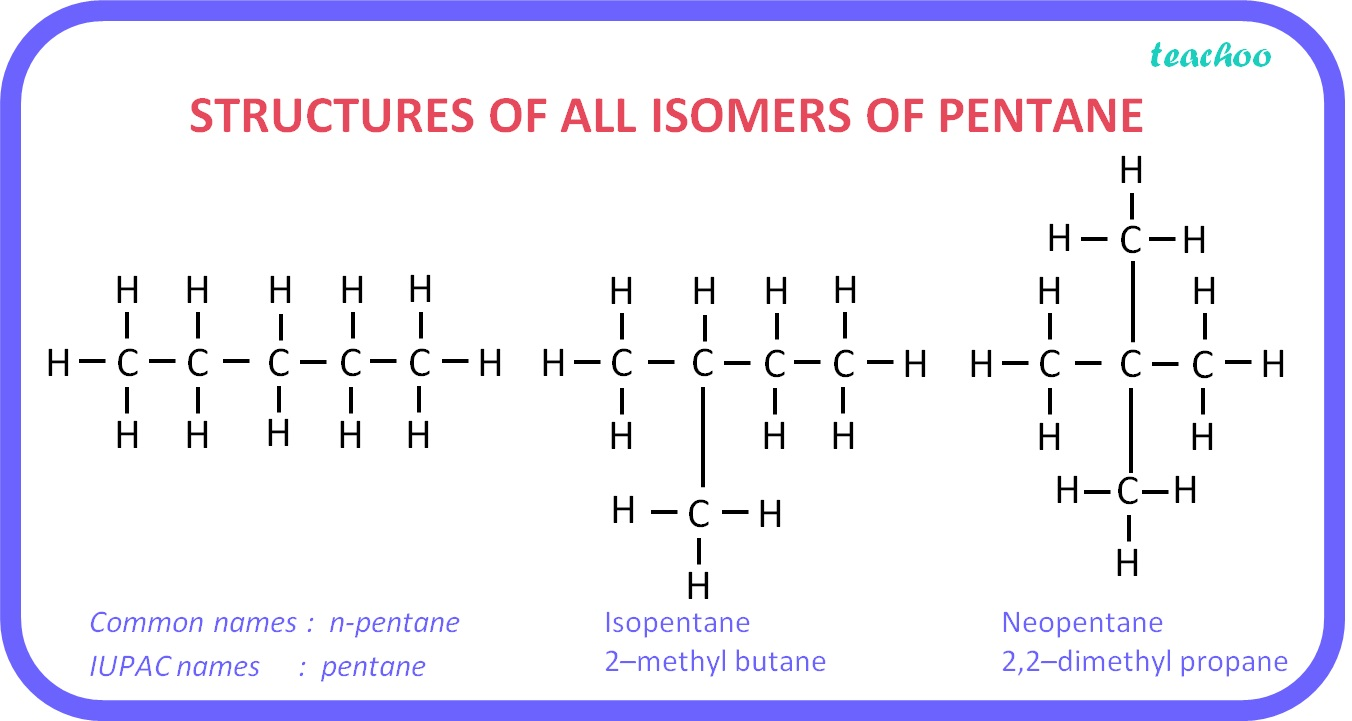### Explanation for n-pentane

• n-Pentane means Normal pentane
• Formula for Normal pentane is C 5 H 12
• ‘pent’ stands for 5, hence it has 5 Carbon atoms
• Number of Hydrogen Atoms = 2 × Carbon Atoms + 2 = 2 × 5 + 2 = 10 + 2 = 12
• Hence we get formula C 5 H 12

In n pentane, we show all 5 carbon atoms together as shown below: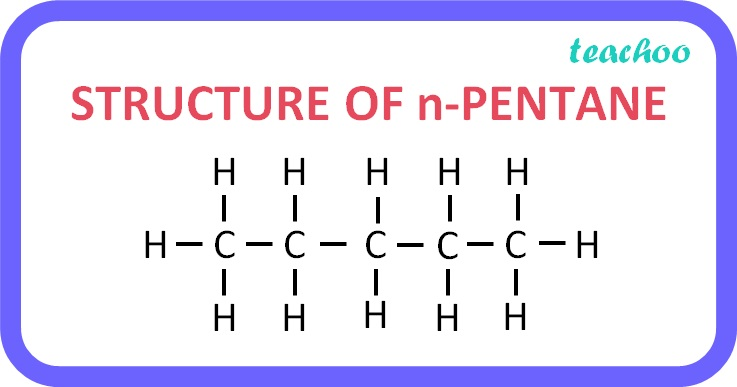### Explanation for isopentane:

• The formula for isopentane is also C 5 H 12 however the arrangement is different
• In this 4 carbon atoms are in a chain and 1 carbon atom forms a branch at the 2nd carbon of the chain. The structure of isopentane is as follows: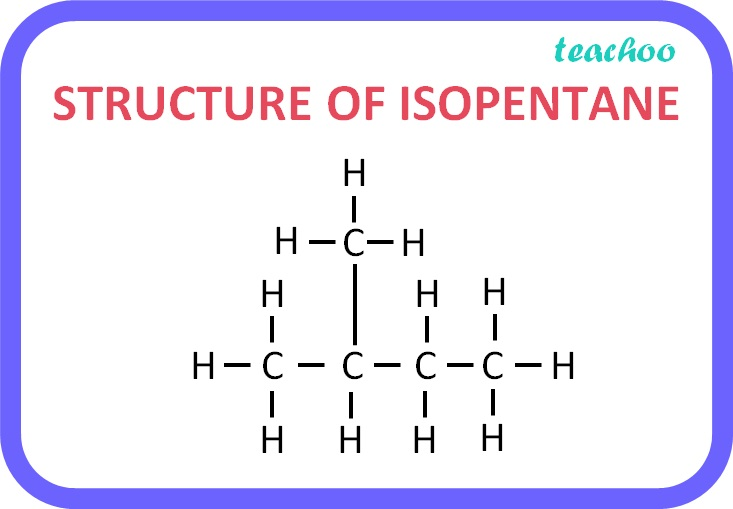### Explanation for neopentane:

• The formula for neopentane is also C 5 H 12 , but the arrangement is different.
• In this 3 carbon atoms form the chain and the remaining 2 form branches on the second carbon atom of the chain as shown in the figure below: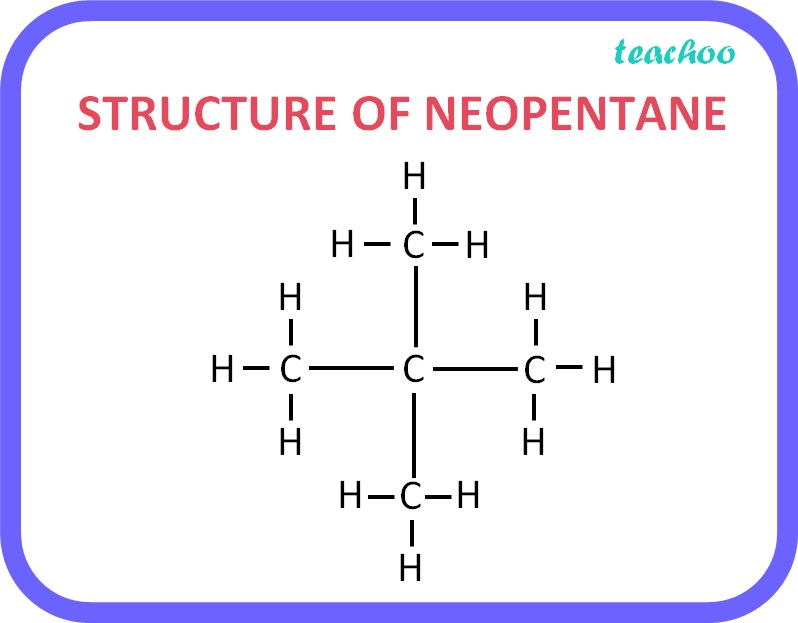Learn in your speed, with individual attention - Teachoo Maths 1-on-1 Class